# Solving MultiStep Equations Objectives to solve equations involving

• Slides: 7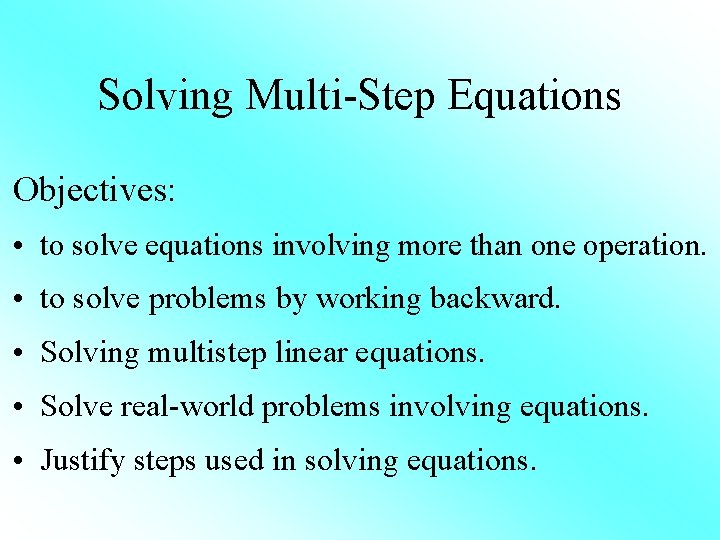Solving Multi-Step Equations Objectives: • to solve equations involving more than one operation. • to solve problems by working backward. • Solving multistep linear equations. • Solve real-world problems involving equations. • Justify steps used in solving equations.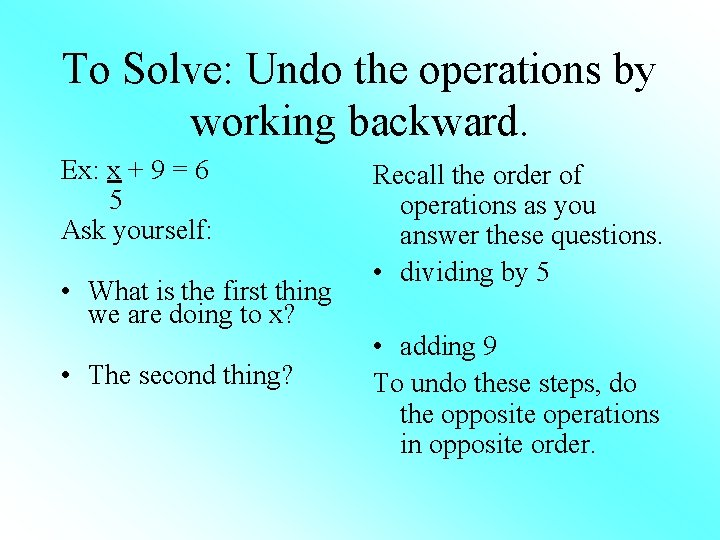To Solve: Undo the operations by working backward. Ex: x + 9 = 6 5 Ask yourself: • What is the first thing we are doing to x? • The second thing? Recall the order of operations as you answer these questions. • dividing by 5 • adding 9 To undo these steps, do the opposite operations in opposite order.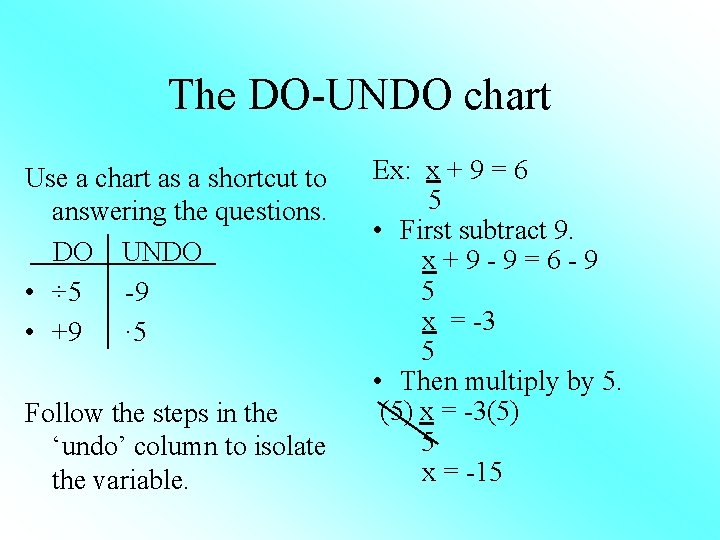The DO-UNDO chart Use a chart as a shortcut to answering the questions. DO UNDO • ÷ 5 -9 • +9 · 5 Follow the steps in the ‘undo’ column to isolate the variable. Ex: x + 9 = 6 5 • First subtract 9. x+9 -9=6 -9 5 x = -3 5 • Then multiply by 5. (5) x = -3(5) 5 x = -15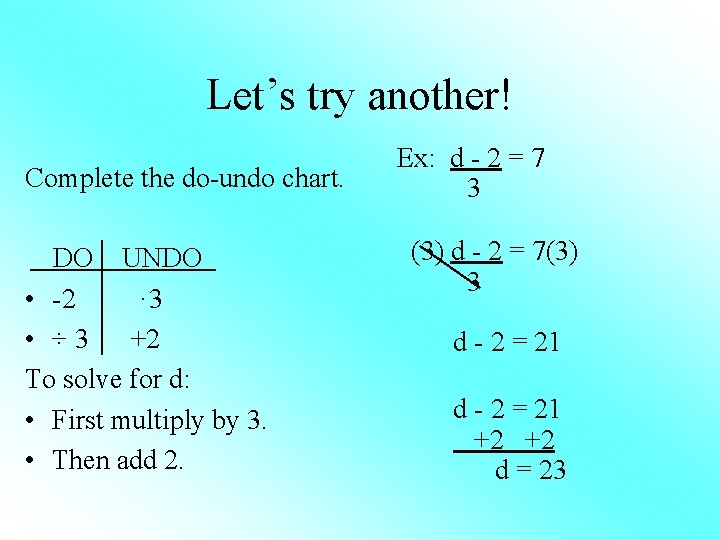Let’s try another! Complete the do-undo chart. DO UNDO • -2 · 3 • ÷ 3 +2 To solve for d: • First multiply by 3. • Then add 2. Ex: d - 2 = 7 3 (3) d - 2 = 7(3) 3 d - 2 = 21 +2 +2 d = 23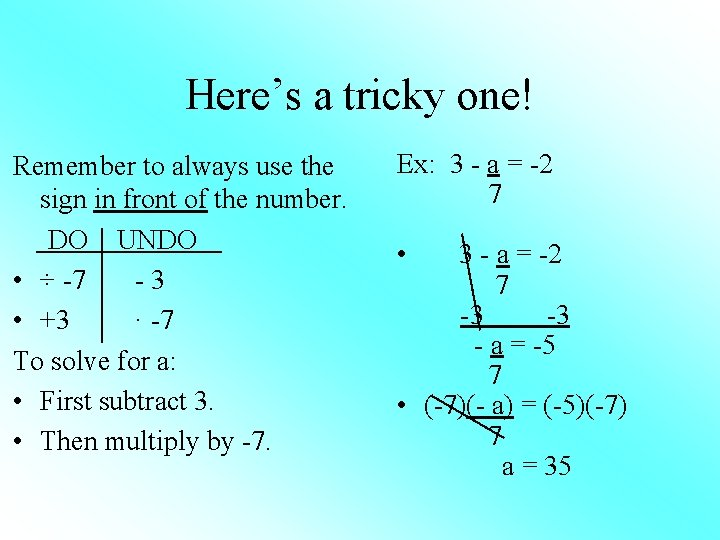Here’s a tricky one! Remember to always use the sign in front of the number. DO UNDO • ÷ -7 -3 • +3 · -7 To solve for a: • First subtract 3. • Then multiply by -7. Ex: 3 - a = -2 7 • 3 - a = -2 7 -3 -3 - a = -5 7 • (-7)(- a) = (-5)(-7) 7 a = 35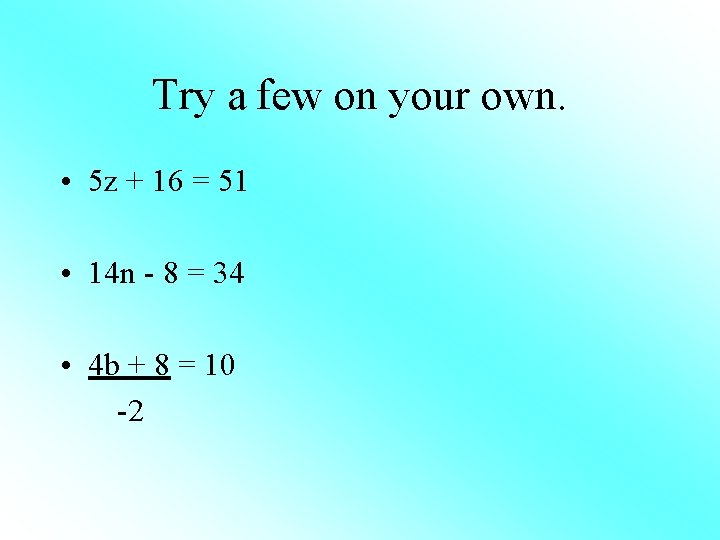Try a few on your own. • 5 z + 16 = 51 • 14 n - 8 = 34 • 4 b + 8 = 10 -2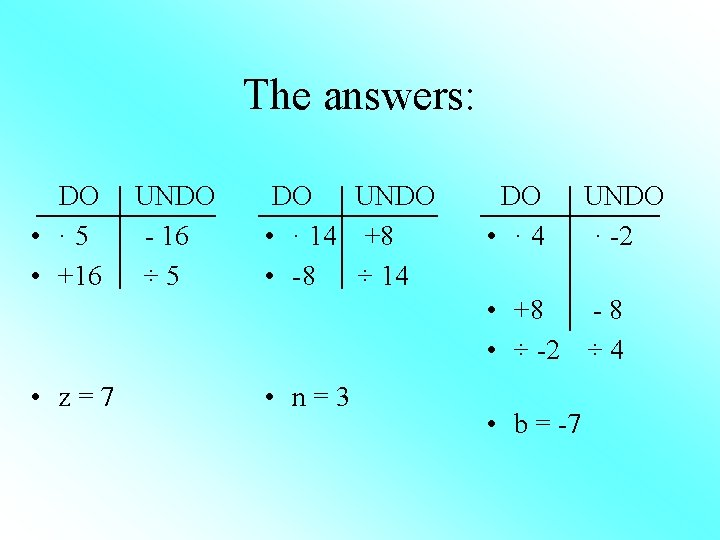The answers: DO • · 5 • +16 UNDO - 16 ÷ 5 DO UNDO • · 14 +8 • -8 ÷ 14 DO • · 4 UNDO · -2 • +8 -8 • ÷ -2 ÷ 4 • z=7 • n=3 • b = -7